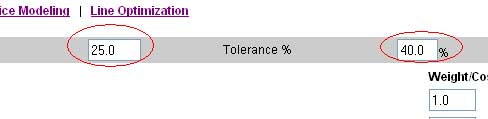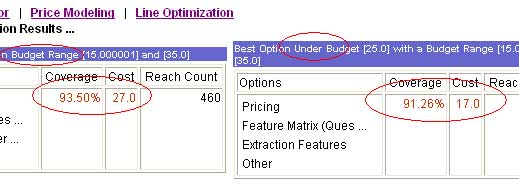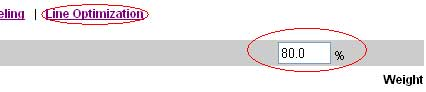# Advanced TURF Analysis Price Modeling

## Advanced TURF Analysis Price Modeling and Line Optimization

TURF Analysis

Price Modeling and Line Optimization analysis can be used in addition to the TURF Analysis and Simulator tools, to optimize the cost and pricing structures. It can be used to answer questions like:

• I have a budget of 5000\$ -- what are the "Optimal" outlets I should advertise on?
• What is the cheapest combination of items that will give me an Unduplicated reach or coverage of 80%.
• What happens if I go 10% over/under budget? What kind of Market share will I gain or loose?

If you are not familiar with the TURF analysis please read introduction to TURF Analysis -- Total Unduplicated Reach and Frequency.

The Simulation tools are essentially part of the TURF Analysis and Simulation tools with the concept of "Cost" or "Price" integrated into each of the options. Since the analysis is relative, you can specify costs or weights in any format that you want. This could be absolute costs, or relative weights. The "budget" can also be specified either as absolute or relative values to the cost.

The TURF Price Modeling and Line Optimization tools can be accessed in the "View Results" -> "Advanced Analysis" -> "TURF Analysis"

There are two modes you can operate the TURF Price Model and Line Optimization Simulator. If you have a fixed (approximately) budget and want to find out what kind of a reach will that budget buy you - Use the "Price Model Simulator". If you have a variable budget, but would like to achieve a minimum "Reach" then use the "Line Optimization Simulator". You can easily flip between both modes and try out different combinations for each of the parameters and see the simulation results.

Price Model Simulator
Given a budget range (budget - tolerance) to (budget + tolerance) the Price Model Simulator will find out every combination of items that will fit into the budget range. The results are then sorted by "Reach" and displayed. This gives you a good indication of kind of "Reach" this price range will get you.

A couple of other results are also displayed after the simulation:

1. Best Option Under Budget - This is the best "Reach" you can get if you strictly wanted to be under-budget.
2. Optimal Reach Option - This is the most "optimal" configuration for this budget range.

Line Optimization Simulator
Use this simulation model if you have requirements for a minimum reach percentage. For example, if you had to reach 85% of the audience, what is the cheapest configuration you can do that? The Line Optimization Simulator takes in just one parameter - the minimum reach percentage. It then tries and simulates every combination of items that match the minimum reach criteria. The results are then sorted by price or cost. The configuration that is the "Cheapest" above the reach % is then chosen and displayed.

Example Illustration:
As part of our data gathering process of what people are looking for when they come to our website, we have a popup survey that asks for what users are looking for:Figure 1

Now we have 7 options here and each of them may have unequal "weights". For example we think that explaining details about Conjoint Analysis is more difficult than explaining our Pricing structure. We may also think that Data Integration Services are equally as difficult as Conjoint Analysis. In order to capture this, we associate a "weight" or a "cost" with each of the options.

Once we have weights associated with each of the options, it's time to play with the "Budget" we have in mind. The budget is simply how much are we willing to "pay" for an optimal configuration. The total cost for all the options here is 67. We estimated a budget of 25, with a "tolerance" of 40%. So we will try to simulate every option that costs between 15 (25 - 40% of 25) to 35 (25 + 40% of 25).Figure 2

The simulation results with these parameters give us the following results:Figure 3

Here we have two comparative results. In the budget range of 15 to 35 the best coverage we can get is 93.5%. However, the best coverage Under the budget of 25 is 91.26%, with a cost of 17.

So, if 91.26% reach is an acceptable coverage rate, then we can easily discount the other alternative, since the cost on that (27) is much higher than one under budget (17)

You can change the budget as well as the "tolerance" on the budget and keep repeating the process as many times as you want to.

Now, let us take the same example and run it through the "Line Optimization" Simulator. The Line Optimization Simulator requires a "minimum" coverage that you are trying to reach. Let us try to find out what is our cheapest solution if we only wanted a reach of 80%.Figure 4

The results of the Line Optimization Simulator: -

Pricing and Feature Matrix here cost us only 6 and gives us the required reach at the lowest cost.

The Price Modeling and Line Optimization simulation tools can be used with any question that accepts multiple choices, much like the TURF Analysis and Simulator tools. These tools can be used for varied purposes and are an easy mechanism to do Cost/Benefit Analysis before making business decisions.

Once again, be careful in assigning weights and costs associated with each of the options. This affects the overall outcome of the analysis. The more accurate the costs are the more realistic the resulting analysis will be. We suggest that you put some thought in deciding the relative cost of each of the items in the option list.